# Proc Freq Explained with Examples

This tutorial explains how to use PROC FREQ with various examples. The PROC FREQ is one of the most frequently used SAS procedures which helps to summarize categorical variable. It calculates count/frequency and cumulative frequency of categories of a categorical variable. In other words, it returns the number and percentage of cases falling in multiple categories of a categorical variable. It's not just restricted to counts. It also produces bar charts and tests for association between two categorical variables.

Create a sample data set

The program below creates a sample SAS dataset which would be used in further examples to explain PROC FREQ.
data example1;
input x y \$ z;
cards;
6 A 60
6 A 70
2 A 100
2 B 10
3 B 67
2 C 81
3 C 63
5 C 55
;
run;
The created dataset looks like below -
X Y Z
6 A 60
6 A 70
2 A 100
2 B 10
3 B 67
2 C 81
3 C 63
5 C 55

Example 1 : To check the distribution of a categorical variable (Character)

Suppose you want to see the frequency distribution of variable 'y'.
proc freq data = example1;
tables y;
run;
The TABLES statements tells SAS to return n-way frequency and crosstabulation tables and computes the statistics for these tables.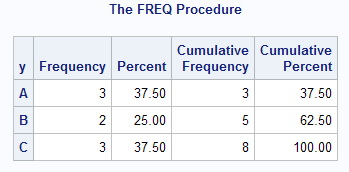Output : PROC FREQ

It answers a question 'which category holds the maximum number of cases'. In this case, the category 'C' contains maximum number of values.

Tip :
Categorical variables are of two types - Nominal and Ordinal. A nominal variable is a categorical variable in which categories do not have any order. For example, gender, city etc. An ordinal categorical variable has categories that can be ordered in a meaningful way. For example, rank, status (high/medium/low) etc.

Example 2 : To remove unwanted statistics in the table

Suppose you do not want cumulative frequency and cumulative percent to be displayed in the table. The option NOCUM tells SAS to not to return cumulative scores.
proc freq data = example1;
tables y /nocum;
run;NOCUM Option

If you want only frequency, not percent distribution and cumulative statistics.
proc freq data = example1;
tables y /nopercent nocum;
run;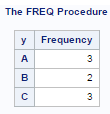NOPERCENT and NOCUM option

Example 3 : Cross Tabulation ( 2*2 Table)

Suppose you want to see the distribution of variable 'y' by variable 'x'.
proc freq data = example1;
tables y * x;
run;Proc Freq Output
The output of the above SAS program is shown in the image above.

Example 4 : Show Table in List Form

Suppose you do not want output to be shown in tabular form. Instead, you want final analysis to be displayed in list form (See the image below)PROC FREQ List Form
proc freq data = example1;
tables y * x / list;
run;
The forward slash followed by LIST keyword produces the list styled table.

Example 5 : Hide Unwanted Statistics in Cross Tabulation
proc freq data = example1;
tables y * x / norow nocol nopercent;
run;

The NOROW option hides row percentage in cross tabulation. Similarly, NOCOL option suppresses column percentage.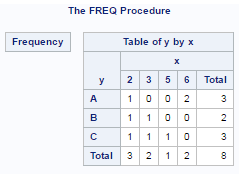NOROW and NOCOL Options

Example 6 : Request Multiple Tables

Suppose you want to generate multiple crosstabs. To accomplish it, you can run the command below-
proc freq data = example1;
tables y * (x z) / norow nocol nopercent;
run;
The tables y*(x z) statement is equivalent to tables y*x y*z statement. In this case, it returns two tables - y by x and y by z.
Example - tables (a b)*(c d); is equivalent to tables a*c  b*c  a*d  b*d;

Example 7 : Number of Distinct Values

The NLEVELS option is used to count number of unique values in a variable.
proc freq data = example1 nlevels;
tables y;
run;
In this case, it returns 3 for variable Y.

Example 8 : Use WEIGHT Statement

The WEIGHT statement is used when we already have the counts. It makes PROC FREQ use count data to produce frequency and crosstabulation tables.
Data example2;
input pre \$ post \$ count;
cards;
Yes Yes 30
Yes No 10
No Yes 40
No No 20
;
run;
proc freq data=example2;
tables pre*post;
weight count;run;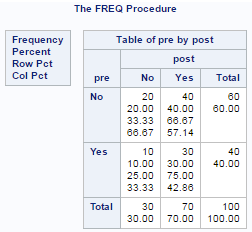PROC FREQ Weight Statement

Example 9 : Store result in a SAS dataset

Suppose you wish to save the result in a SAS dataset instead of printing it in result window.
proc freq data = example1 noprint;
tables y *x / out = temp;
run;
The OUT option is used to store result in a data file. NOPRINT option prevents SAS to print it in results window.

Example 10 : Run Chi-Square Analysis

The CHISQ option provides chi-square tests of homogeneity or independence and measures of association between two categorical variables.  Also it helps to identify the statistically significant categorical variables that we should include in our predictive model. All the categorical variables with a chi-square value less than or equal to 0.05 are kept.
proc freq data = example1 noprint;
tables y * x/chisq;
output All out=temp_chi chisq;
run;
Example 11 : Generate Bar Chart and Dot Plot

The bar chart can be generated with PROC FREQ. ​To produce a bar chart for variable 'y', the plots=freqplot (type=bar) option is added. By default, it shows frequency in graph. In order to show percent, you need to add scale=percent. The ODS graphics ON statement tells SAS to produce graphs. Later we turn it off.
Ods graphics on;Proc freq data=example1 order=freq;
Tables y/ plots=freqplot (type=bar scale=percent);
Run;
Ods graphics off;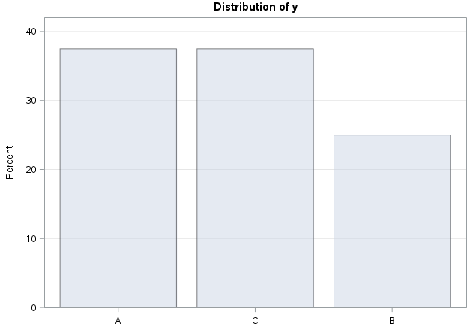Bar Chart with PROC FREQ

Similarly, we can produce dot plot by adding type=dot. See the implementation below-
Ods graphics on;
Proc freq data=example1 order=freq;
Tables y/ plots=freqplot (type=dot);
Run;
Ods graphics off;

Example 12 : Include Missing Values in Calculation

By default, PROC FREQ does not consider missing values while calculating percent and cumulative percent. The number of missing values are shown separately (below the table). Refer the image below.
Proc freq data=sashelp.heart;
Tables deathcause;
Run;Exclude Missing : Proc FREQ
By adding MISSING option, it includes missing value as a separate category and all the respective statistics are generated based on it.
Proc freq data=sashelp.heart;
Tables deathcause / missing;
Run;Include Missing : PROC FREQ

Example 13 : Ordering / Sorting

In PROC FREQ, the categories of a character variable are ordered alphabetically by default. For numeric variables, the categories are ordered from smallest to largest value.

To sort categories on descending order by frequency (from largest to smallest count), add ORDER=FREQ option
Proc freq data=sashelp.heart order = FREQ;
Tables deathcause / missing;
Run;
It is generally advisable to show distribution of a nominal variable after sorting categories by frequency. For ordinal variable, it should be shown based on level of categories.

To order categories based on a particular FORMAT, you can use order = FORMATTED option.

Conclusion

PROC FREQ is a simple but powerful SAS procedure. This tutorial was designed for beginners who have no background of any programming language. Hope the above examples help to understand the procedure crystal clear.Share
Related PostsDeepanshu founded ListenData with a simple objective - Make analytics easy to understand and follow. He has over 10 years of experience in data science. During his tenure, he has worked with global clients in various domains like Banking, Insurance, Private Equity, Telecom and Human Resource.

34 Responses to "Proc Freq Explained with Examples"
1.Good explaination!

1.2.Great tutorial...
could you also explain more on 'WEIGHT statement'

1.yes youre right? even i do not understand this syntax called 'weight';

3.Good work, Keep it up :)

1.2.Good question bank and explanations.Keep posting!!

4.Simply amazing...

5.superb description

6.Good Work, luck to stumble upon your site.

7.Amazing tutorial. Keep up the good work!

8.Amazing description. You are really making it easy for SAS beginners :)
Thank you for your effort and resources

9.how to use by statement in proc freq

10.Best...I learned alot

11.12.Good presentation sir

13.Hi Deepanshu,
Well explained. I have 1 query regarding ods file. We are using VM space and when we are trying to save the following error occured. Could you please help.
ods rtf file = "C:\Users\kiran\table1a.rtf" style = forNESUG;
ERROR: Insufficient authorization to access /opt/sasinside/SASConfig/Lev1/SASApp/C:\Users\kiran\table1a.rtf.

14.Excellent explanations. Really easy to understand SAS programming through your portal.

15.Excellent explanations. Really easy to understand SAS programming through your portal.

16.17.Very good!!

18.Excellent explanations. You make me the life easier.

19.This comment has been removed by the author.

20.That is a very nice and well explained summary, thank you!

21.thanks for excellent turorials. copy and pasted your code but not working..

proc freq data = example1 noprint;
tables y * x/chisq;
output All out=temp_chi chisq;
run;

22.Fantastic! Every one of the tutorials worked for me.
Thank you very much.

Charles

1.23.Thank you for you explanation. Very nice of you!

24.Great, thanks

25.Exellent explanation ! Thank You so much!

26.This website is pure gold for newcomers like me who want to get into coding.
Thank you so very much!!

27.Good work 👍

28.write a program to get output using proc freq
X1 X2
N 19
M 10
F 9

29.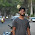write a program to get output using proc freq
X1 X2
N 19
M 10
F 9

Ans: I think we can create a new data set and run the proc freq. I pasted the code below I hope this is what you expecting please let me know.

data temp;
input x1 :\$10. x2;
datalines;
N 19
M 10
F 9
;
run;
proc freq data= temp;
run;

Next → ← Prev